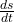## If we ignore air resistance, a falling body will fall 16t2 feet in t seconds. What is the average velocity between t

Question

If we ignore air resistance, a falling body will fall 16t2 feet in t seconds. What is the average velocity between t

in progress 0
6 months 2021-08-01T11:20:05+00:00 1 Answers 25 views 0

262.4 m/s

Explanation:

The complete question is

If we ignore air resistance, a falling body will fall 16t^2 feet in t seconds. What is the average velocity between t=8 and t=8.4? Round your answer to two decimal places if necessary.

The distance fallen s = 16t^2

The velocity v == 32t

If we substitute the values of t into the velocity v, we’ll have

at t = 8 s,     V1 = 32 x 8 = 256 m/s

at t = 8.4 s,  V2 = 32 x 8.4 = 268.8 m/s

Average velocity = (V2 – V1)/2 = (268.8 + 256)/2 = 262.4 m/s